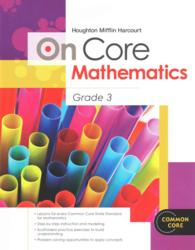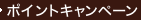•### On Core Mathematics Grade 3 (Houghton Mifflin Harcourt Math Common Core) （CSM WKB）

• 在庫がございません。海外の書籍取次会社を通じて出版社等からお取り寄せいたします。
通常6～9週間ほどで発送の見込みですが、商品によってはさらに時間がかかることもございます。
重要ご説明事項
1. 納期遅延や、ご入手不能となる場合がございます。
2. 複数冊ご注文の場合、分割発送となる場合がございます。
3. 美品のご指定は承りかねます。
• ≪洋書のご注文につきまして≫ 「海外取次在庫あり」および「国内仕入れ先からお取り寄せいたします」表示の商品でも、納期の目安期間内にお届けできないことがございます。あらかじめご了承ください。

• 製本 Paperback:紙装版/ペーパーバック版／ページ数 210 p.
• 言語 ENG,ENG
• 商品コード 9780547575230
• DDC分類 512

### Table of Contents

`Operations and Algebraic Thinking      Represent and solve problems involving      multiplication and division    Lesson 1 CC.3.OA.1 Count Equal Groups          1  (2)    Lesson 2 CC.3.OA.1 Algebra · Relate     3  (2)    Addition and Multiplication    Lesson 3 CC.3.OA.2 Size of Equal Groups        5  (2)    Lesson 4 CC.3.OA.2 Number of Equal Groups      7  (2)    Lesson 5 CC.3.OA.2 Model with Bar Models       9  (2)    Lesson 6 CC.3.OA.3 Skip Count on a Number      11 (2)    Line    Lesson 7 CC.3.OA.3 Model with Arrays           13 (2)    Lesson 8 CC.3.OA.3 Multiply with 2 and 4       15 (2)    Lesson 9 CC.3.OA.3 Multiply with 5 and 10      17 (2)    Lesson 10 CC.3.OA.3 Multiply with 3 and 6      19 (2)    Lesson 11 CC.3.OA.3 Problem Solving            21 (2)    · Model Division    Lesson 12 CC.3.OA.3 Algebra · Relate    23 (2)    Subtraction and Division    Lesson 13 CC.3.OA.3 Investigate ·       25 (2)    Model with Arrays    Lesson 14 CC.3.OA.3 Divide by 2                27 (2)    Lesson 15 CC.3.OA.3 Divide by 5                29 (2)    Lesson 16 CC.3.OA.4 Algebra · Find      31 (2)    Unknown Factors    Lesson 17 CC.3.OA.4 Divide by 8                33 (2)      Understand properties of multiplication      and the relationship between      multiplication and division    Lesson 18 CC.3.OA.5 Algebra ·           35 (2)    Commutative Property of Multiplication    Lesson 19 CC.3.OA.5 Algebra ·           37 (2)    Multiply with 1 and 0    Lesson 20 CC.3.OA.5 Algebra ·           39 (2)    Distributive Property    Lesson 21 CC.3.OA.5 Algebra ·           41 (2)    Associative Property of Multiplication    Lesson 22 CC.3.OA.5 Algebra ·           43 (2)    Division Rules for 1 and 0    Lesson 23 CC.3.OA.6 Algebra · Relate    45 (2)    Multiplication and Division      Multiply and divide within 100    Lesson 24 CC.3.OA.7 Multiply with 7            47 (2)    Lesson 25 CC.3.OA.7 Multiply with 8            49 (2)    Lesson 26 CC.3.OA.7 Multiply with 9            51 (2)    Lesson 27 CC.3.OA.7 Algebra · Write     53 (2)    Related Facts    Lesson 28 CC.3.OA.7 Divide by 10               55 (2)    Lesson 29 CC.3.OA.7 Divide by 3                57 (2)    Lesson 30 CC.3.OA.7 Divide by 4                59 (2)    Lesson 31 CC.3.OA.7 Divide by 6                61 (2)    Lesson 32 CC.3.OA.7 Divide by 7                63 (2)    Lesson 33 CC.3.OA.7 Divide by 9                65 (2)      Solve problems involving the four      operations, and identify and explain      patterns in arithmetic    Lesson 34 CC.3.OA.8 Problem Solving            67 (2)    · Model Addition and Subtraction    Lesson 35 CC.3.OA.8 Problem Solving            69 (2)    · Model Multiplication    Lesson 36 CC.3.OA.8 Problem Solving            71 (2)    · Multiplication    Lesson 37 CC.3.OA.8 Problem Solving            73 (2)    · Two-Step Problems    Lesson 38 CC.3.OA.8 Investigate ·       75 (2)    Order of Operations    Lesson 39 CC.3.OA.9 Algebra · Number    77 (2)    Patterns    Lesson 40 CC.3.OA.9 Algebra ·           79 (2)    Patterns on the Multiplication Table    Lesson 41 CC.3.OA.9 Algebra ·           81 (2)    Describe Patterns  Number and Operations in Base Ten      Use place value understanding and      properties of operations to perform      multi-digit arithmetic    Lesson 42 CC.3.NBT.1 Round to the Nearest      83 (2)    Ten or Hundred    Lesson 43 CC.3.NBT.1 Estimate Sums             85 (2)    Lesson 44 CC.3.NBT.1 Estimate Differences      87 (2)    Lesson 45 CC.3.NBT.2 Mental Math Strategies    89 (2)    for Addition    Lesson 46 CC.3.NBT.2 Algebra · Use      91 (2)    Properties to Add    Lesson 47 CC.3.NBT.2 Use the Break Apart       93 (2)    Strategy to Add    Lesson 48 CC.3.NBT.2 Use Place Value to Add    95 (2)    Lesson 49 CC.3.NBT.2 Mental Math Strategies    97 (2)    for Subtraction    Lesson 50 CC.3.NBT.2 Use Place Value to        99 (2)    Subtract    Lesson 51 CC.3.NBT.2 Combine Place Values      101(2)    to Subtract    Lesson 52 CC.3.NBT.3 Problem Solving           103(2)    · Use the Distributive Property    Lesson 53 CC.3.NBT.3 Multiplication            105(2)    Strategies with Multiples of 10    Lesson 54 CC.3.NBT.3 Multiply Multiples of     107(2)    10 by 1-Digit Numbers  Number and Operations -- Fractions      Develop understanding of fractions as      numbers    Lesson 55 CC.3.NF.1 Equal Parts of a Whole     109(2)    Lesson 56 CC.3.NF.1 Equal Shares               111(2)    Lesson 57 CC.3.NF.1 Unit Fractions of a        113(2)    Whole    Lesson 58 CC.3.NF.1 Fractions of a Whole       115(2)    Lesson 59 CC.3.NF.1 Fractions of a Group       117(2)    Lesson 60 CC.3.NF.1 Find Part of a Group       119(2)    Using Unit Fractions    Lesson 61 CC.3.NF.1 Problem Solving            121(2)    · Find the Whole Group Using Unit    Fractions    Lesson 62 CC.3.NF.2a Fractions on a Number     123(2)    Line      CC.3.NF.2b    Lesson 63 CC.3.NF.3a Investigate ·      125(2)    Model Equivalent Fractions    Lesson 64 CC.3.NF.3b Equivalent Fractions      127(2)    Lesson 65 CC.3.NF.3c Relate Fractions and      129(2)    Whole Numbers    Lesson 66 CC.3.NF.3d Problem Solving           131(2)    · Compare Fractions    Lesson 67 CC.3.NF.3d Compare Fractions with    133(2)    the Same Denominator    Lesson 68 CC.3.NF.3d Compare Fractions with    135(2)    the Same Numerator    Lesson 69 CC.3.NF.3d Compare Fractions         137(2)    Lesson 70 CC.3.NF.3d Compare and Order         139(2)    Fractions  Measurement and Data      Solve problems involving measurement and      estimation of intervals of time, liquid      volumes, and masses of objects    Lesson 71 CC.3.MD.1 Time to the Minute         141(2)    Lesson 72 CC3.MD.1 A.M. and P.M.               143(2)    Lesson 73 CC.3.MD.1 Measure Time Intervals     145(2)    Lesson 74 CC.3.MD.1 Use Time Intervals         147(2)    Lesson 75 CC.3.MD.1 Problem Solving            149(2)    · Time Intervals    Lesson 76 CC.3.MD.2 Estimate and Measure       151(2)    Liquid Volume    Lesson 77 CC.3.MD.2 Estimate and Measure       153(2)    Mass    Lesson 78 CC.3.MD.2 Solve Problems About       155(2)    Liquid Volume and Mass      Represent and interpret data    Lesson 79 CC.3.MD.3 Problem Solving            157(2)    · Organize Data    Lesson 80 CC.3.MD.3 Use Picture Graphs         159(2)    Lesson 81 CC.3.MD.3 Make Picture Graphs        161(2)    Lesson 82 CC.3.MD.3 Use Bar Graphs             163(2)    Lesson 83 CC.3.MD.3 Make Bar Graphs            165(2)    Lesson 84 CC.3.MD.3 Solve Problems Using       167(2)    Data    Lesson 85 CC.3.MD.4 Use and Make Line Plots    169(2)    Lesson 86 CC.3.MD.4 Measure Length             171(2)      Geometric measurement: understand      concepts of area and relate area to      multiplication and to addition    Lesson 87 CC.3.MD.5 Understand Area            173(2)      CC.3.MD.5a    Lesson 88 CC.3.MD.5b Measure Area              175(2)      CC.3.MD.6    Lesson 89 CC.3.MD.7a Use Area Models           177(2)    Lesson 90 CC.3.MD.7b Problem Solving           179(2)    · Area of Rectangles    Lesson 91 CC.3.MD.7c Area of Combined          181(2)    Rectangles      CC.3.MD.7d      Geometric measurement: recognize      perimeter as an attribute of plane      figures and distinguish between linear      and area measures    Lesson 92 CC.3.MD.8 Investigate ·       183(2)    Model Perimeter    Lesson 93 CC.3.MD.8 Find Perimeter             185(2)    Lesson 94 CC.3.MD.8 Algebra · Find      187(2)    Unknown Side Lengths    Lesson 95 CC.3.MD.8 Same Perimeter,            189(2)    Different Areas    Lesson 96 CC.3.MD.8 Same Area, Different       191(2)    Perimeters  Geometry      Reason with shapes and their attributes    Lesson 97 CC.3.G.1 Describe Plane Shapes       193(2)    Lesson 98 CC.3.G.1 Describe Angles in Plane    195(2)    Shapes    Lesson 99 CC.3.G.1 Identify Polygons           197(2)    Lesson 100 CC.3.G.1 Describe Sides of          199(2)    Polygons    Lesson 101 CC.3.G.1 Classify Quadrilaterals    201(2)    Lesson 102 CC.3.G.1 Draw Quadrilaterals        203(2)    Lesson 103 CC.3.G.1 Describe Triangles         205(2)    Lesson 104 CC.3.G.1 Problem Solving            207(2)    · Classify Plane Shapes    Lesson 105 CC.3.G.2 Investigate ·       209    Relate Shapes, Fractions, and Area`